# JEE Main 2020 Question Paper with Solution (Jan 8th first shift)

## JEE Main 2020 Question Paper with Solutions, Morning Session ( 9:30 am to 12:30 pm)

National Test Agency( NTA) Is conducting JEE Mains 2020 Exam in two shifts, through an online mode. JEE Main 2020 is a computer-based test. In this article, we are going to share a JEE main 2020 question paper with solutions for the morning session of 8th January 2020. These solutions are prepared by experts. We suggest you go through the article once, it will help you to understand your performance and with the help of this article, you will be able to understand that which concept is important, Type of exam questions, etc.

JEE Main 2020 exam - Chemistry ( 8th January first shift)

Q1. The number of chiral carbons in penicillin.

Solution:

As shown in the figure below, there are 3 chiral carbons in penicillin.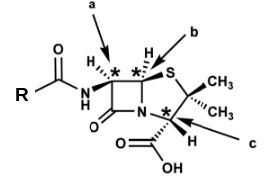Q2. The correct order for ionization enthalpy for the elements Na, Mg, Al and Si

Solution:

The correct ionization enthalpy order is given below:

Si > Mg > Al > Na

Q3) What will happen when we will heat gypsum above 393K?

Solution

The reaction occurs as follows:

Gypsum                     Plaster of Paris

Q4) The third ionisation enthalpy of the following elements is minimum for:

(a) Co
(b) Mn
(c) Fe
(d) Ni

Solution

The third ionisation potential data for the given element is given below:

Co3+          -           3237

Mn3+         -           3258

Fe3+          -           2967

Ni3+           -           3400

The electronic configuration of Fe2+ is [Ar]3d6. Thus it is easier to remove one electron to get [Ar]3d5 electronic configuration as it is half-filled and thus it is stable.

Therefore, Option(c) is correct.

Q5) Gases that cause greenhouse effect are:

Solution

The gases that cause greenhouse gases are: CO2, O3 and H2O

Q6) Arrange them in increasing C-O bond length
Phenol, p-methoxyphenol and methanol

Solution

The correct order of bond length is given below:
Phenol < p-methoxyphenol < methanol

Q7) Following vanderwaal forces are present in ethyl acetate liquid:

(a) H-bond, London forces.

(b) dipole-dipole interaction, H-bond

(c) dipole–dipole interaction, London forces

(d) H-bond, dipole-dipole interaction, London forces

Solution

Ethyl acetate is polar molecule so dipole-dipole interaction will be present there

Q8) The complex that can show fac- and mer- isomerism is:

(a) [CoCl2(en)2]
(b) [Co(NH3)4Cl2]+
(c) [Co(NH3)3(NO2)3]+
(d) [Pt(NH3)2Cl2]

Solution

Complexes of the type MA3B3 exist in tow geometrical forms which are named as facial (fac-) and meridional (mer-). Co(NH3)3(NO2)3]+ can be represented in fac- and mer- isomeric forms as follows: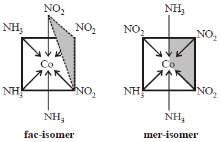Q9)  Number of S–O bond in S2O82– and number of S–S bond in Rhombic sulphur are respectively:

Solution

The structure of S2O82– is given below: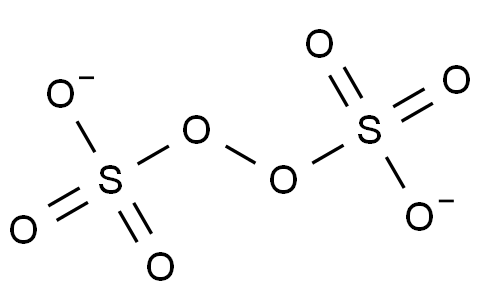Rhombic sulphur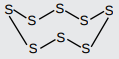Thus, the number of S-S bonds is 8 and the number of S-O bonds is also 8.

Q10) Arrange the following compounds in order of dehydrohalogenation (E1) reaction.

(a)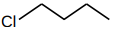(b)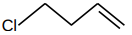(c)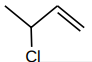(d)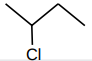Solution

E1 reaction proceeds via carbocation formation, therefore greater the stability of carbocation, faster the E1 reaction

C > D > B > A

Q11) Find the value (in ml) of AgNO3[0.125M] which reacts with 0.3g of [Co(NH3)6]Cl3.

Solution

The reaction occurs as follows:

Molecular weight of [Co(NH3)6]Cl3 = 267.48g/mol

Number of moles of [Co(NH3)6]Cl3 = 0.3/267.48 = 1.125 x 10-3 moles.

1 mole of [Co(NH3)6]Cl3 gives 3 moes of AgCl.

Thus, moles of AgCl = 3 x 1.125 x 10-3 moles

Now, 3 moles of AgNO3 reacts with 3 moles of AgCl

Thus, moles off AgNO3 = 3 x 1.125 x 10-3 moles

Now, volume(L) of AgNO3 = (3 x 1.125 x 10-3)/0.125 = 27 x 10-3 L
volume(in mL) of AgNO3 = 27ml

Q12) Change in activation energy when enzyme is added at T. Rate of reaction of increase 106 times.

(a) -2.303 x 6RT

(b) +6RT

(c) - RT

(d) +2.303 x 6RT

Solution

We know:

Catalyst decrease the activation energy require for a reaction to proceed and thus increase the reaction rate.

Therefore, Option(a) is correct.

Q13) How to calculate the strength of NaOH?

(a) NaOH from burette titrated with conc. H2SO4 from conical flask.
(c) NaOH from burette titrated with oxalic acid from conical flask.

Solution

To calculate the strength of NaOH, it is titrated against oxalic acid.

Thus, Option(C) is correct.

Q14) Which of the following option is incorrect?

(a) Glucose exists in 2 anomeric forms.
(b) Glucose reacts with hydroxylamine to give oxime.
(c) Glucose reacts with Schiff reagent.
(d) Glucose forms pentaacetate.

Solution

Glucose does not react with Schiff reagent because Schiff reagent is used for aldehydes.

Therefore, Option(C) is correct.

Q15) According to Hardy Schultz rule, correct order of flocculation value for Fe(OH)3 sol is :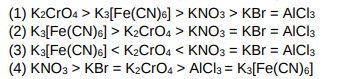Solution

According to hardy-schultz rule,

Q16) Given, for H-atom

Select the correct options regarding this formula for Balmer series.

(A) n1 = 2
(B) Ionization energy of H atom can be calculated from above formula.
(C)  is for n2 = 3.
(D) If  decreases then spectrum lines will converse.

Solution

Since,  so for wavelength to be longest or maximum the energy gap should be minimum. For Balmer series, transition for longest wavelength is from n = 3 to n = 2.

Therefore, Option(c) is correct.

Q17) Select the correct stoichiometry and its ksp value according to given graphs.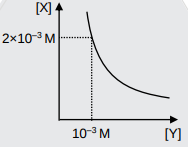(a) XY, Ksp = 2×10–6

(b) XY2, Ksp = 4×10–9

(c) X2Y, Ksp = 9×10–9

(d) XY2, Ksp = 1×10–9

Solution

2 x 10-3            10-3

Ksp = [X+ ] [Y ]

Ksp = 2 × 10–3 × 10–3

Ksp = 2 × 10–6

Therefore, Optioin(a) is correct.

Q18) A gas undergoes expansion according to the following graph. Calculate work done by the gas.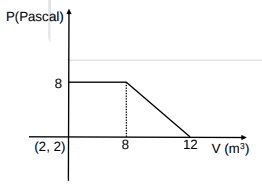Solution

Q19) Calculated the mass of FeSO4.7H2O, which must be added in 100 kg of wheat to get 10 PPM of Fe.

Solution

Fe = 1 gram

56 g of Fe in 1 mol of  FeSO4.7H2O (molar mass 278 gram /mol)

Therefore 1 gram of Fe is present in = (1/56) moles of FeSO4.7H2O

mass of FeSO4.7H2O =

Q20)  Given

Calculate electrode potential at pH = 5.

Solution

Q21) Reagent used for the given conversion is:Solution

B2H6 is very selective and usually used to reduce acid to alcohol.

Q22) Major product in the following reaction is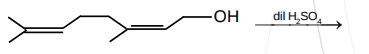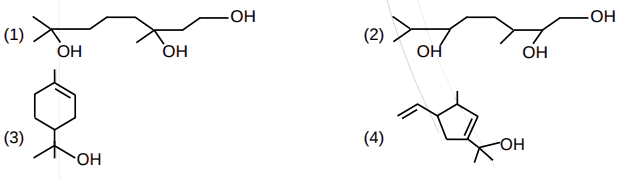SolutionQ23)(a) Intermolecular force of attraction of X > Y
(b) Intermolecular force of attraction of X < Y.
(C) Intermolecular force of attraction of Z < X.

Select the correct option(s).
(a) A and C
(b) A and B
(c) B only
(d) B and C

Solution
At a particular temperature as intermolecular force of attraction increases vapour pressure decreases.

Therefore, Option(c) is correct.

Q24)Product A and B are respectively:

(a)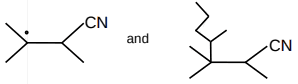(b)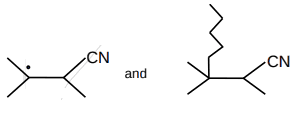(c)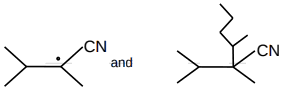(d)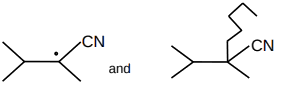Solution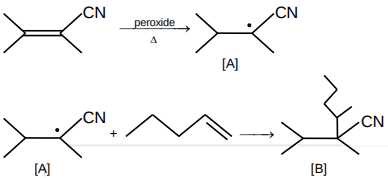[A] is more stable radical and undergoes Markovnikov addition to form [B].

Q25) Two liquids isohexane and 3-methylpentane has boiling point 60°C and 63°C. They can be separated by:

(a) Simple distillation and isohexane comes out first.
(b) Fractional distillation and isohexane comes out first.
(c) Simple distillation and 3-Methylpantane comes out first.
(d) Fractional distillation and 3-Methylpantane comes out first.

Solution

Liquid having lower boiling point comes out first in fractional distillation. Simple distillation can't be used as boiling point difference is very small.

JEE Main 2020 exam - Mathematics (8th January first shift)

Q1. Find the value of

Solution:

Q2. If y(x) is a solution of the differential equation  such that, then

Solution:

Q3. Find the sum

Solution:

Q4. In a bag there are 5 red balls, 3 white balls and 4 black balls. Four balls are drawn from the bag. Find the number of ways of in which at most 3 red balls are selected

Solution:

Q5. No.of 3×3 matrices from the set {-1,0,1} such that is 3

Solution:

Let matrix A

Q6

Solution:

Q7. Normal to curve  at point p  intersects y-axis at Tangent at p has slope m. Find |m|

Solution:

Equation of the normal

Normal intersect at (0,3/2)

Q8.

Solution:

Q9. The equation  has real roots. Find the least possible value of a.

Solution:

least possible value 8.

Q10.

Solution:

Q11. Which of the following is a tautology

Solution:

Q12.  then the minimum value of f(x) is

Solution:

Q13.

Solution:

Q14

Solution: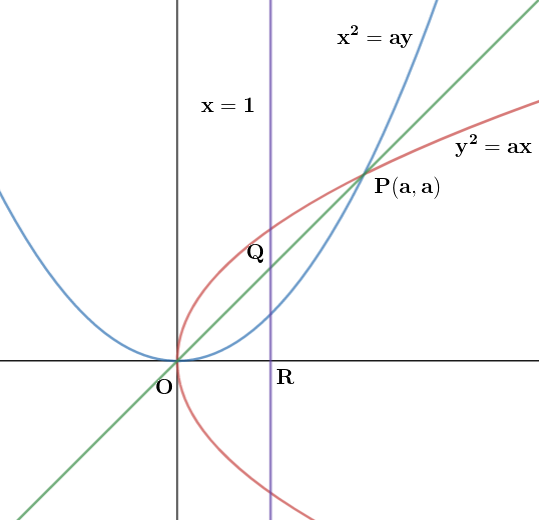By solving above you will get

Q15

Solution:

Q16

Solution:

Q17

Solution:

Q18. Mean and standard deviations of 10 observations are 20 and 2 respectively. If p (p 0) is multiplied to each observation and then q (q  0) is subtracted then new mean and standard deviation becomes half of original value . Then find q

Solution:

If each observation is multiplied with p & then q is subtracted

New mean

and new standard deviations

Q19. If maximum value of  then relation between a, b, c is

Solution

Q20. Let P(A) = 1/3, P(B) = 1/6 where A and B are independent events then

Solution:

A & B are independent events so

Q21. Roots of the equation  lie on the curve , where z is a complex number then

Solution:

Let   be roots of the equation

so

So,

Q22. If the volume of parallelopiped whose their coterminous edges are  and  is 1 cubic unit then cosine of angle between

Solution:

Q23. Shortest distance between the lines  is

Solution:

Q24. Let ABC is a triangle whose vertices are A(1, –1), B(0, 2), C(x', y')and area of ABC is 5 and C(x', y') lie on , then

Q25. The system of equation  is inconsistent, then  can be

Solution:

Hence, it has infinitely many solutions

For non infinite solution

JEE Main 2020 exam - Physics ( 8th January first shift)

Q1. A wind-powered generator converts and energy into electrical energy. Assume that the generator convents a fixed fraction of the wind energy intercepted by to blades into electrical energy for wind speed V, the electrical power output will be proportional to

Solution :

Q2. Two capacitors C1 and C2, when connected in parallel the effective capacitance was found to be 10microFarad. These capacitors, when connected individually to a battery of emf 1V, the energy of C2, was 4 times the energy of C1. The equivalent capacitance of these capacitors, when connected in series, will be?

Solution :

When capacitors are in parallel,

So,

Now when they are individually connected to 1V battery, then

Energy of C2=4 x energy of C1

So, C2=8 and C1=2

So when both are in series, then

Q3. Four resistors of resistance 12 ohms, 4 ohms, 10 ohms, and 15 ohms in the cyclic order are connected to form a wheat bridge. What value of resistance must be connected in parallel to 10-ohm resistance to get a balanced Wheatstone bridge?

Solution:

For balanced Wheatstone bridge

As

So using

We get

let we connected x-ohms in parallel to 10-ohm resistance

i.e

we get

Q4. Express stopping potential V0 in terms of h(planck's constant), G(gravitational constant), c(speed of light) and A(Ampere).

Solution:

Let

Now,

So,

From here we will get: p-q=1---------(1)

2p+3q+r=2----------(2)

-p-2q-r=-3----------(3)

s=-1-----------(4)

From equation 1, 2,3 and 4 we will get: p=0,q=-1, r=5 and s=-1

So,

Q5. A potentiometer wire of 1200 cm is connected with emf of 5V and 20 ohms (internal resistance). The null point is 1000 cm and the current flowing through the wire is 60mA. Find the resistance of the potentiometer wire.

options

1) 100-ohm b) 10-ohm c) 20-ohm d) 50-ohm

Solution:

In the potentiometer, a battery of known emf  E is connected in the secondary circuit. A constant current I is flowing through AB from driver circuit . The jockey is slide on potentiometer wire AB to obtain null deflection in the galvanometer. Let   be the length at which galvanometer shows null deflection.

Since the potential of wire AB (V) is proportional to the length AB(L).

Similarly

So we getgiven current through potentiometer wire =60mA

V=iR

Q6. A plano concave lens has a refractive index of 1.5 and radius of convex part is 30 cm. Find tthe focal length of the system.

Solution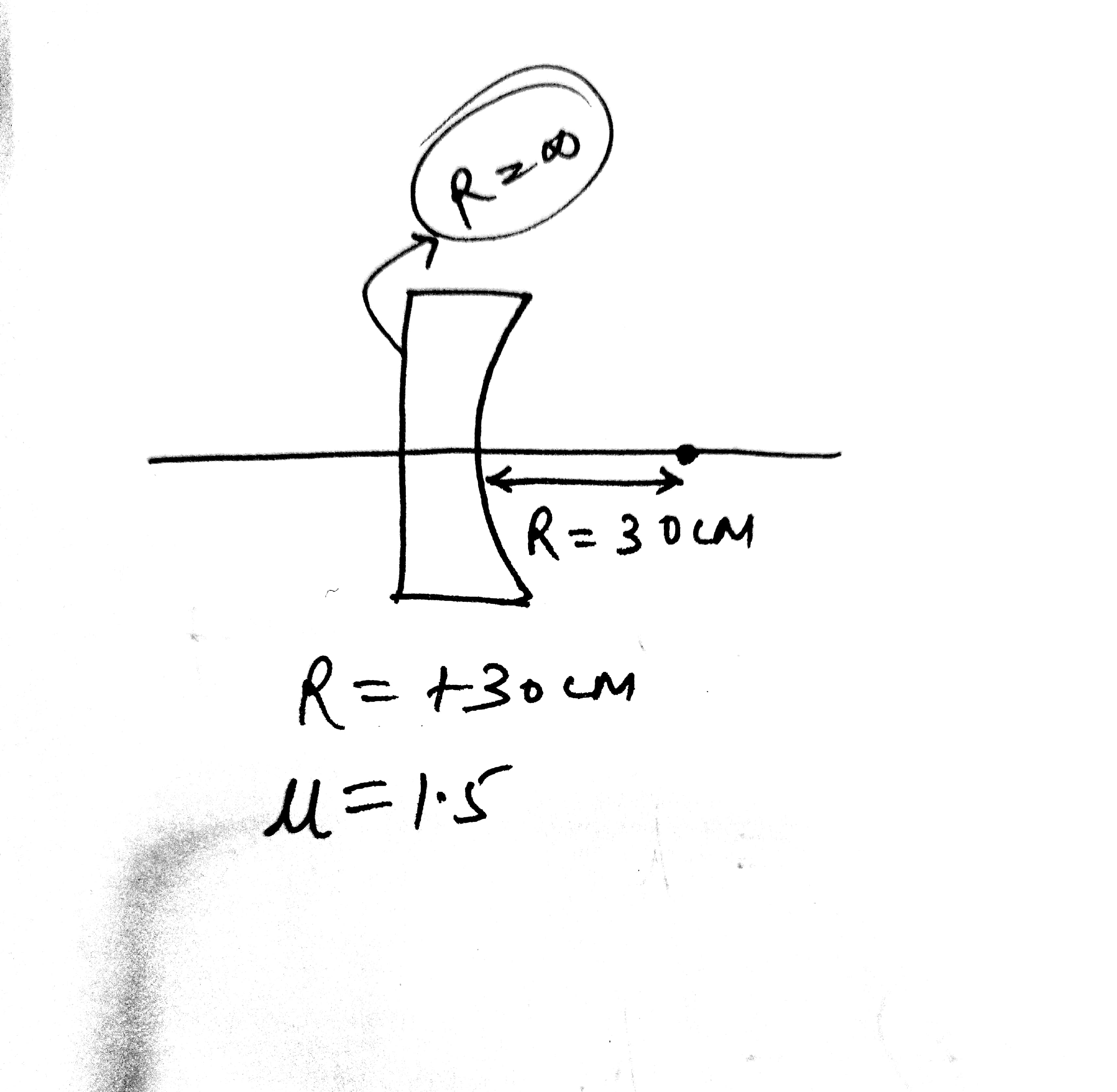the focal length of the lens is given as

So,

So f= -60 cm

Q7. The magnifying power of the telescope of tube length 60cm is 5  for the normal settings. Then what is its focal length of the eyepiece

a) 30
b )10
c )20
d )40

Solution

Q8. A block of mass m is connected at one end of spring fixed at other end having natural length and spring constant K. The block is rotated with constant angular speed in gravity free space. Find the elongation in spring.

SolutionAs natural lentgh=l0

Let elongation=x

Mass m is moving with angular velocity  in a radius r

where

Due to elongation x spring force is given by

And

as

So

Q9.  3 charges are placed in a circle of radius 'd' as shown in figure. Find the electric field along x-axis at centre of circle.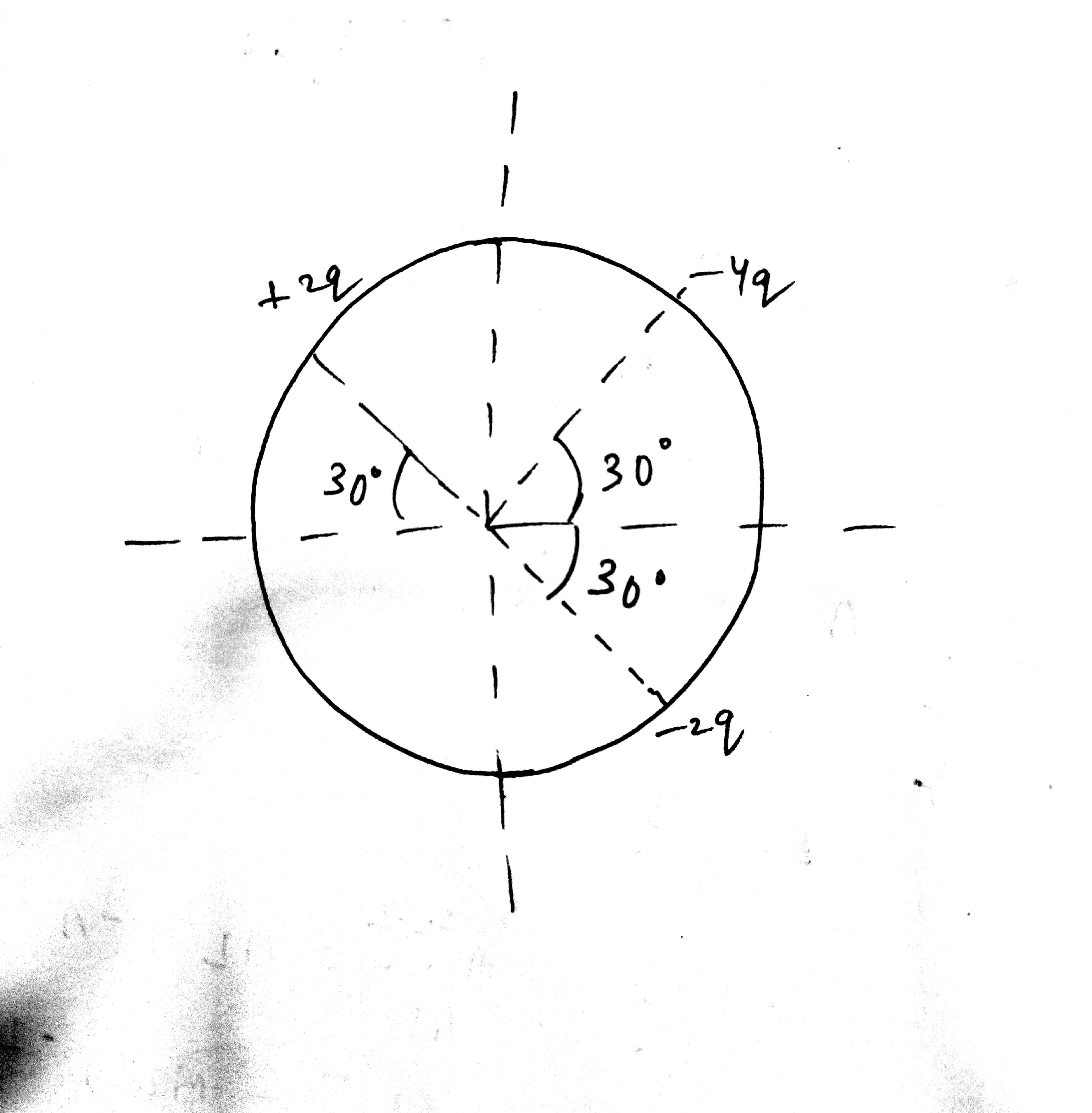Solution

Let E1 be the resultant electric field due to charge 2q and - 2q. The resultant E1 is in the direction of -2q

E2 due to -4q is in the direction of -4q and magnitude is given by

The net electric field in the x direction is

Q10. Select the P vs V graph corresponding to the given V vs T graph:-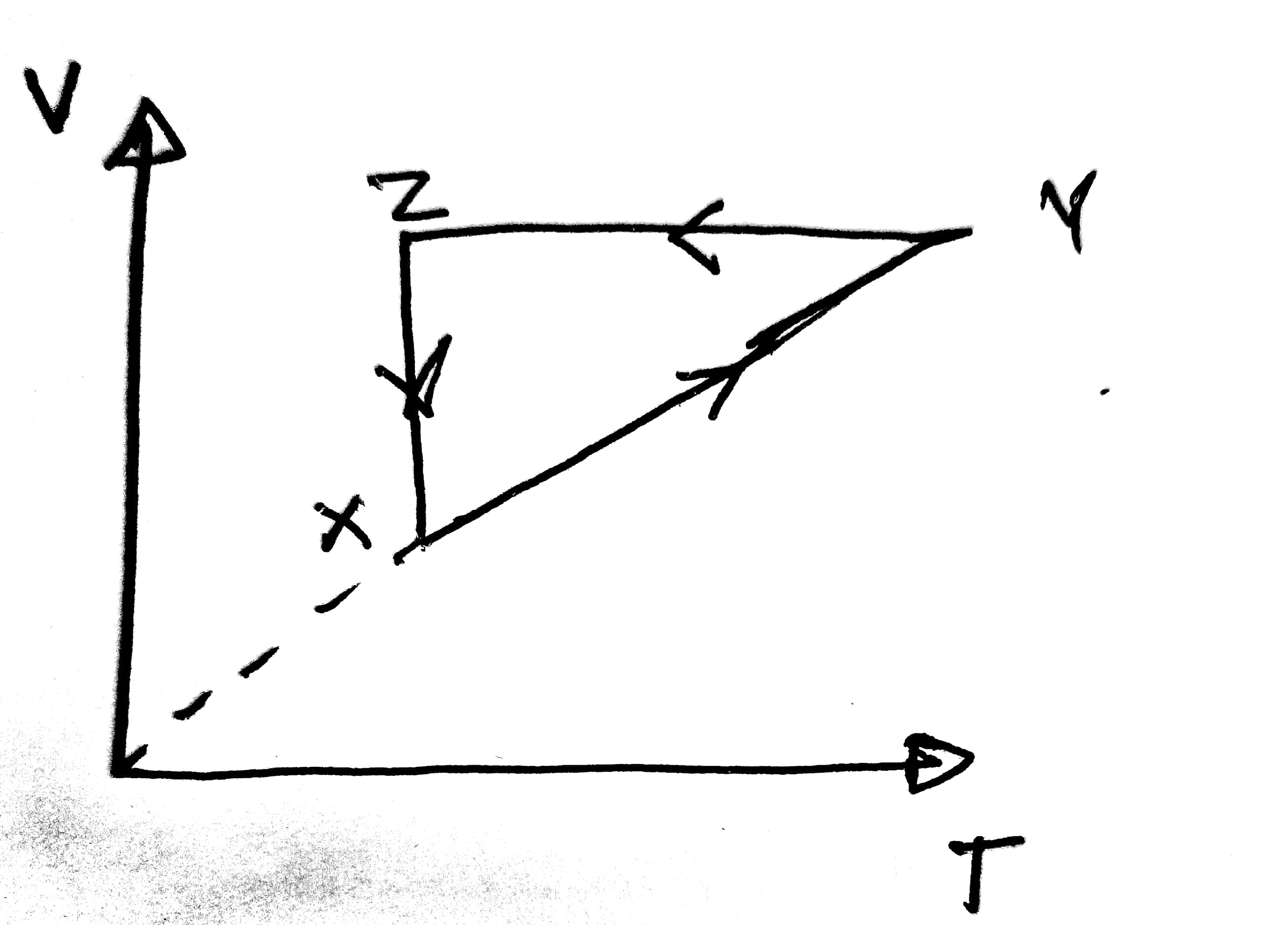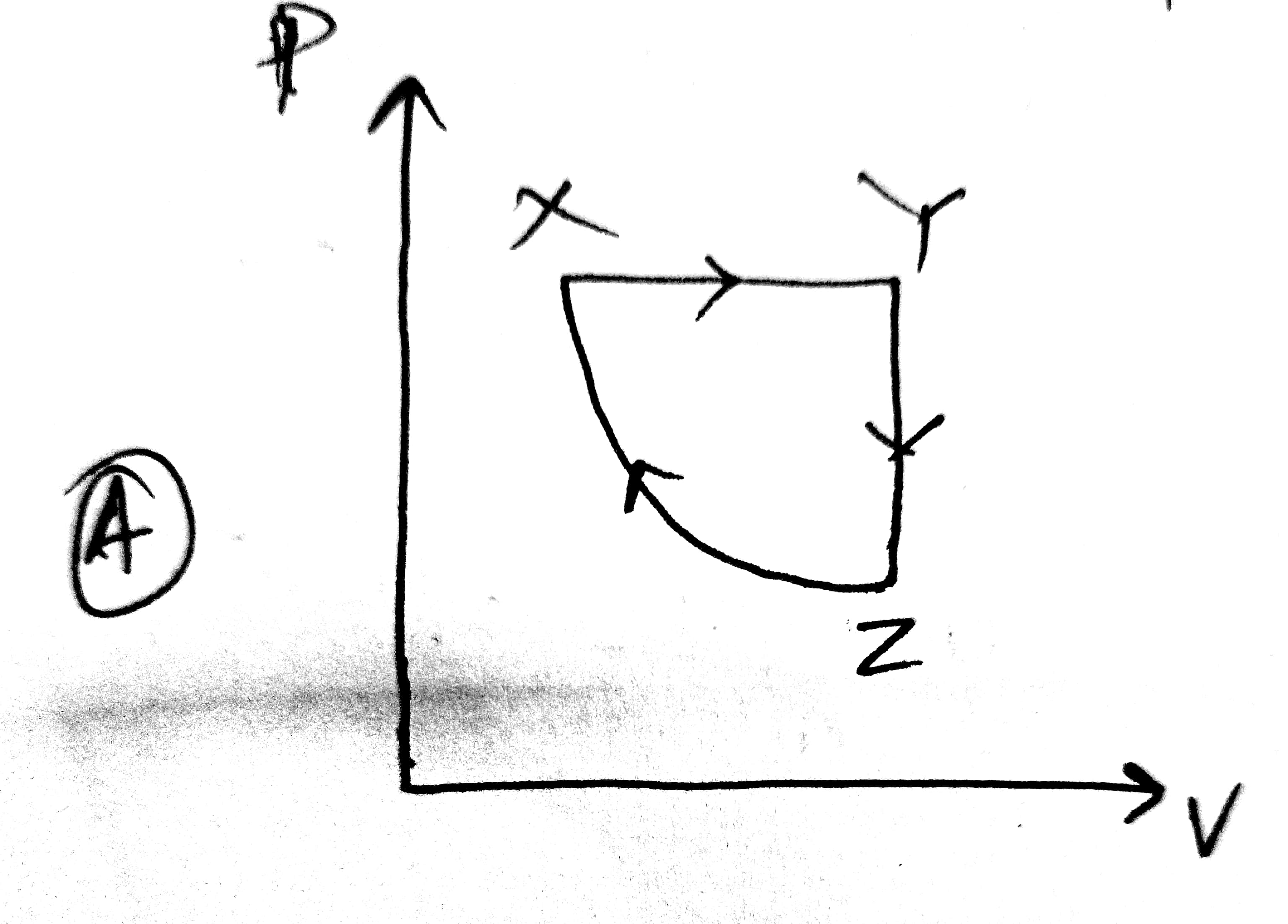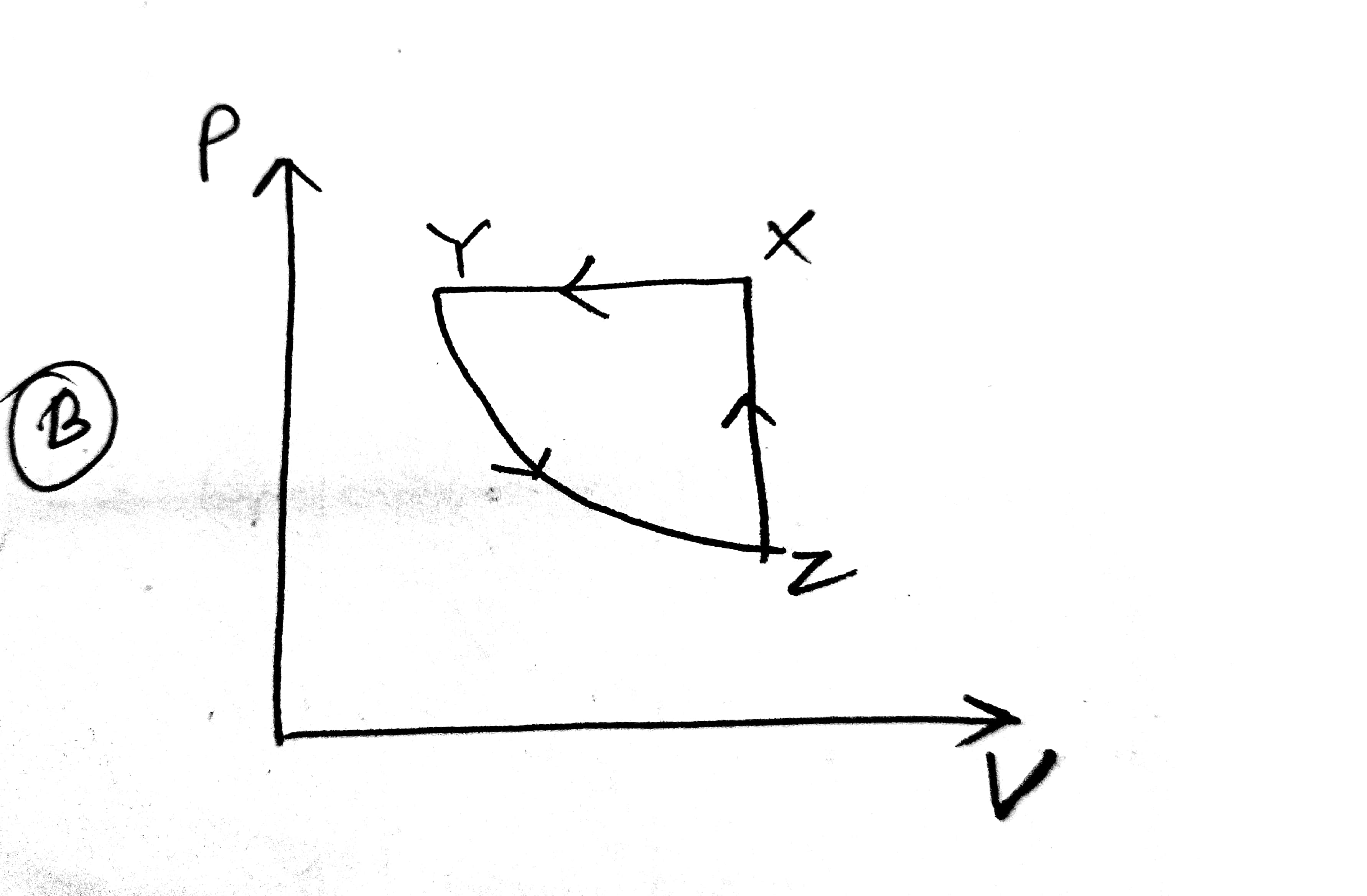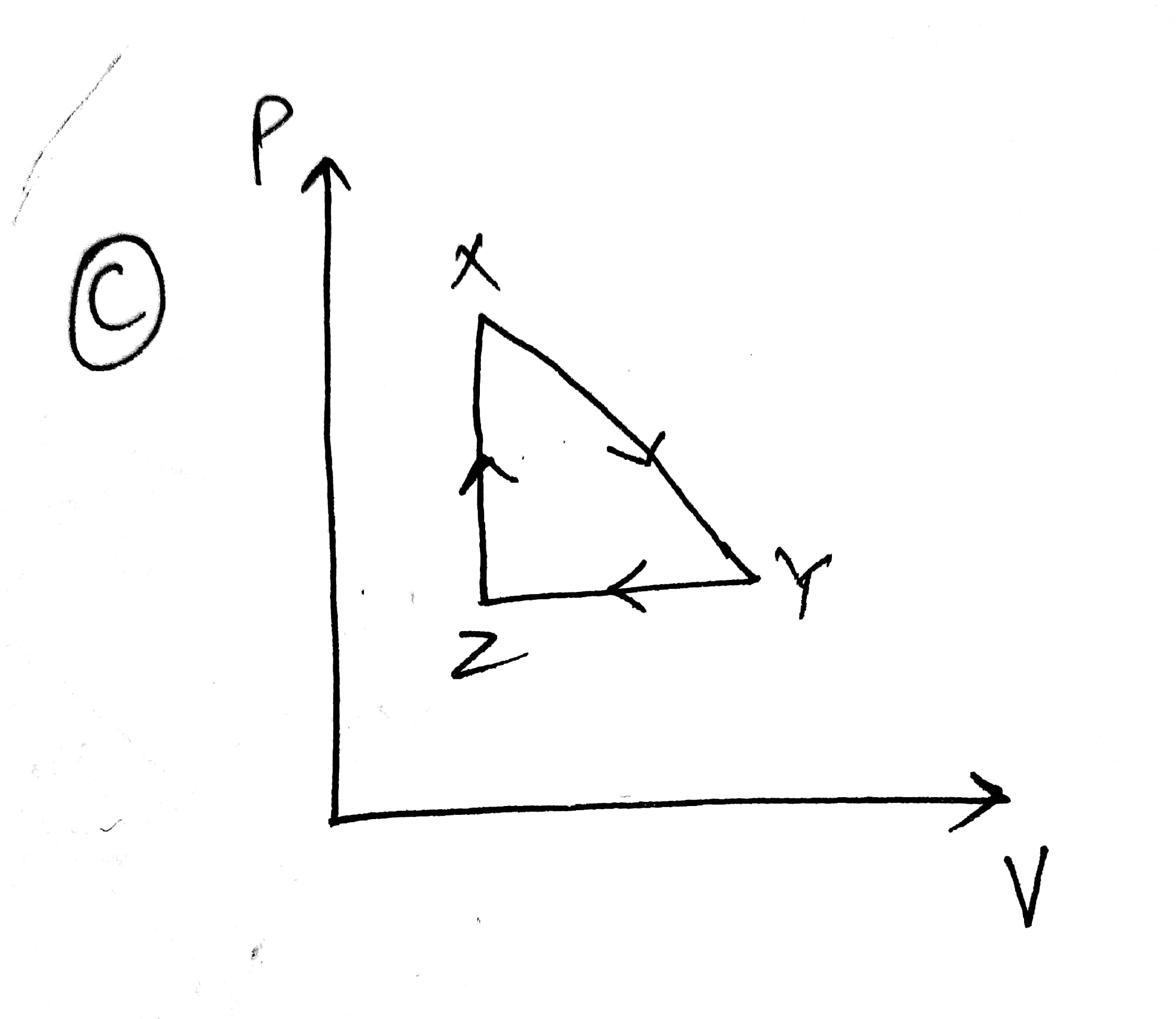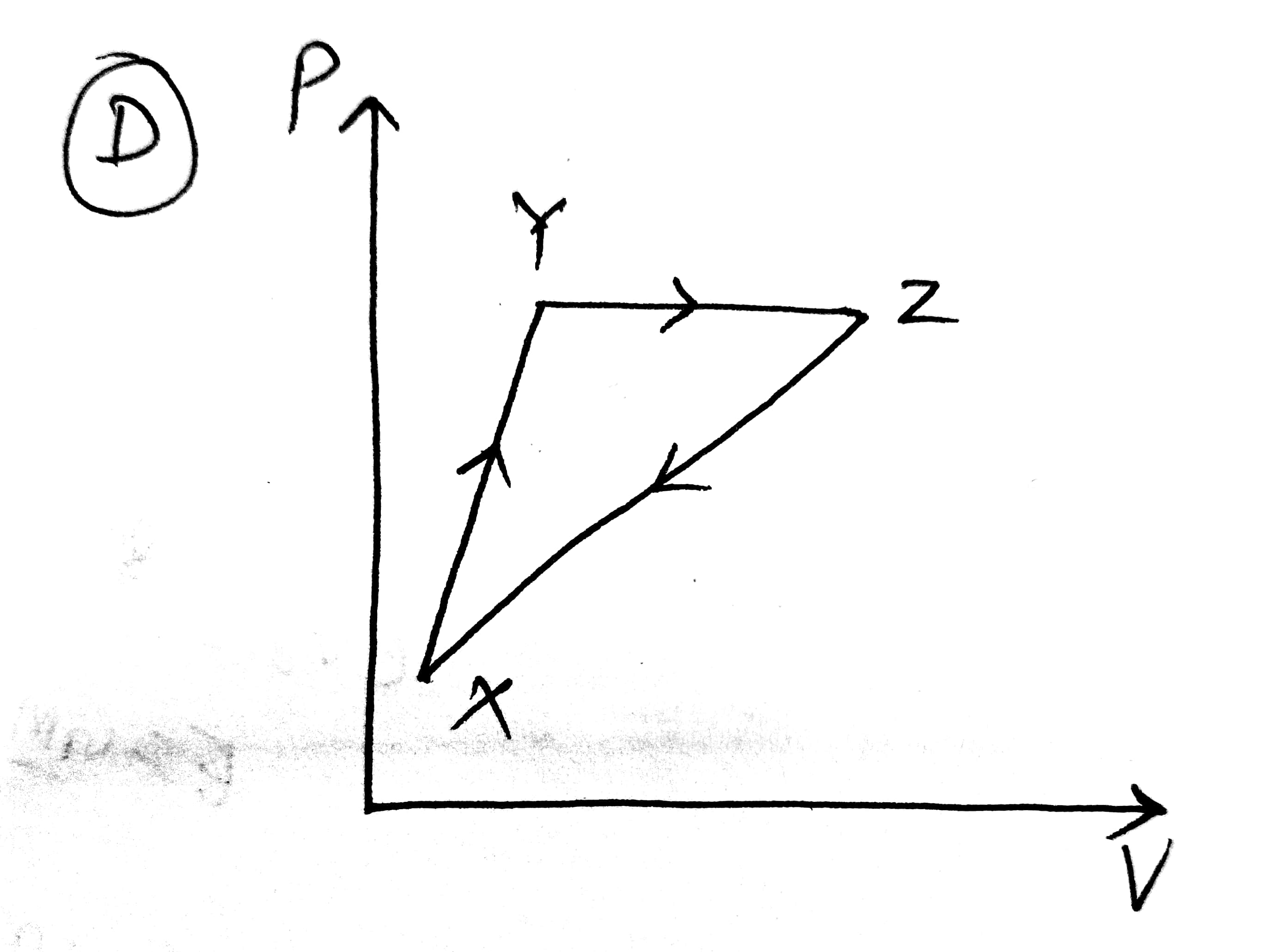Solution

Since xy is passing through origin. So,

So for ideal gas : P= constant

Now Y-Z is constant volume graph (from question). Since Z-X is constant temperature grapg it is hyperbolic in nature. So option 1 is correct

Q11.  Find the co-ordinates of centre of mass of the lamina shown in figure:-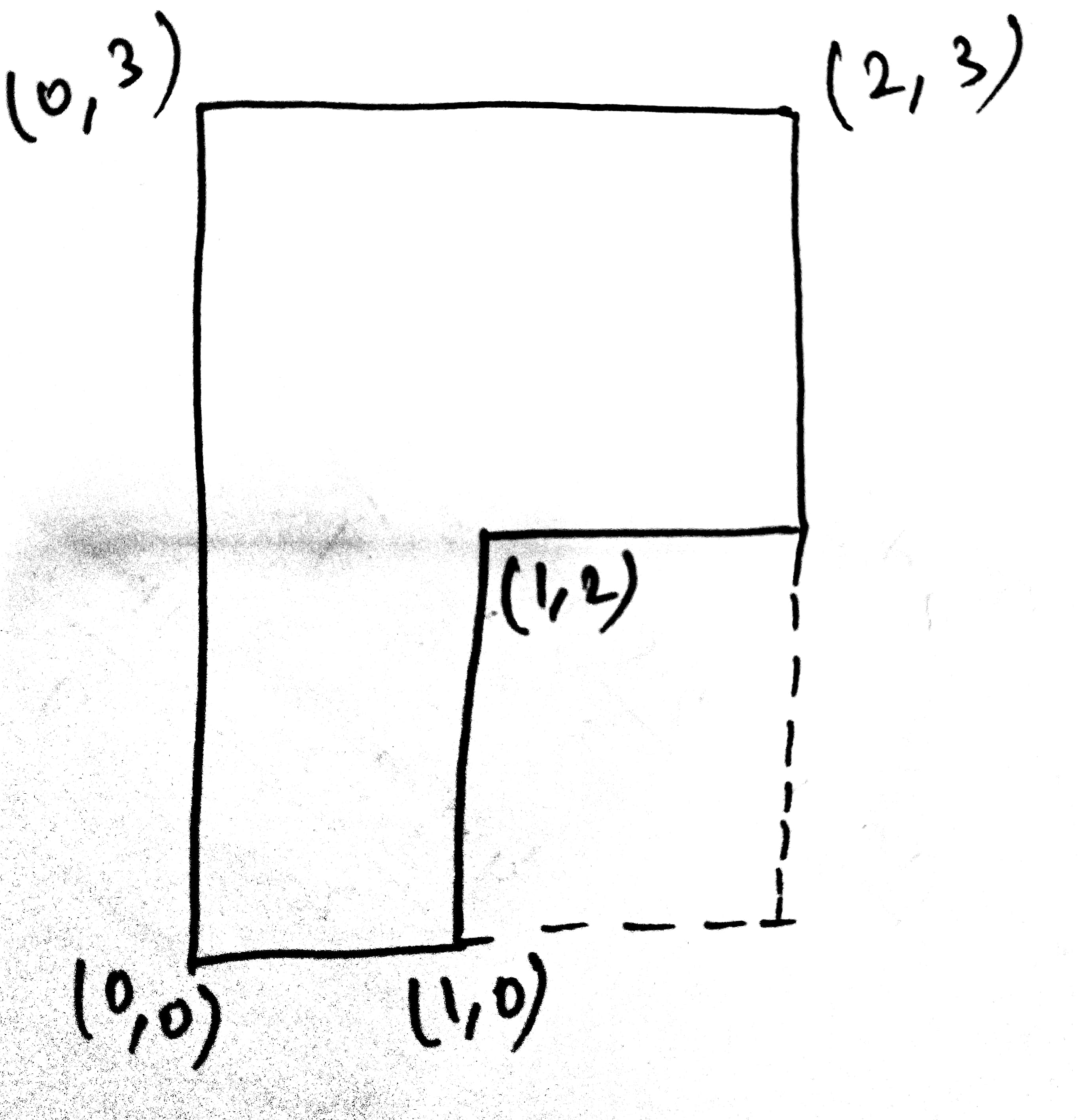Solution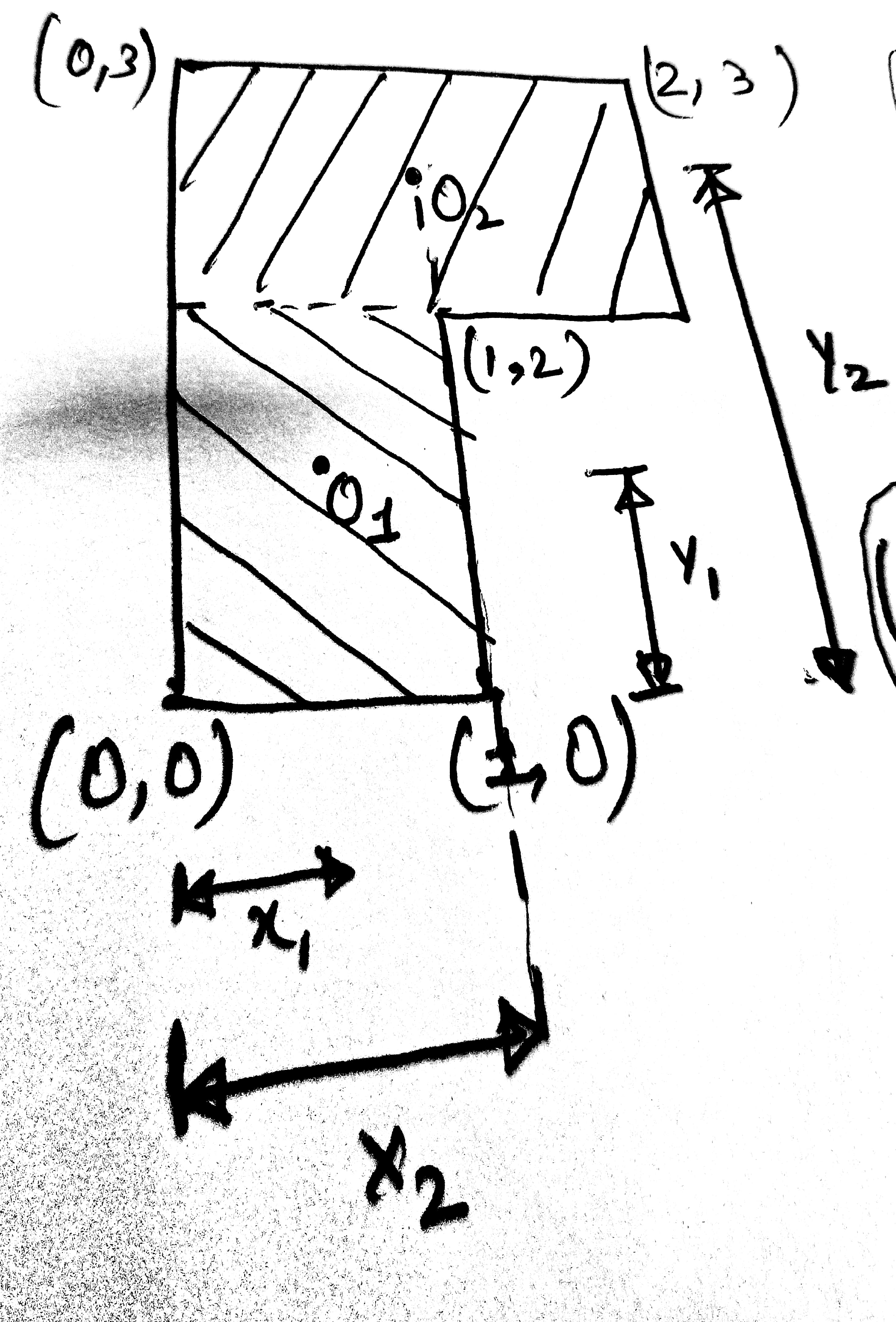The co-ordinate of O1 is (0.5,1),  O2 is (1, 2.5)

Q12. Which graph correctly represents variation between relaxation time (t) of gas molecules with absolute temperature of gas T.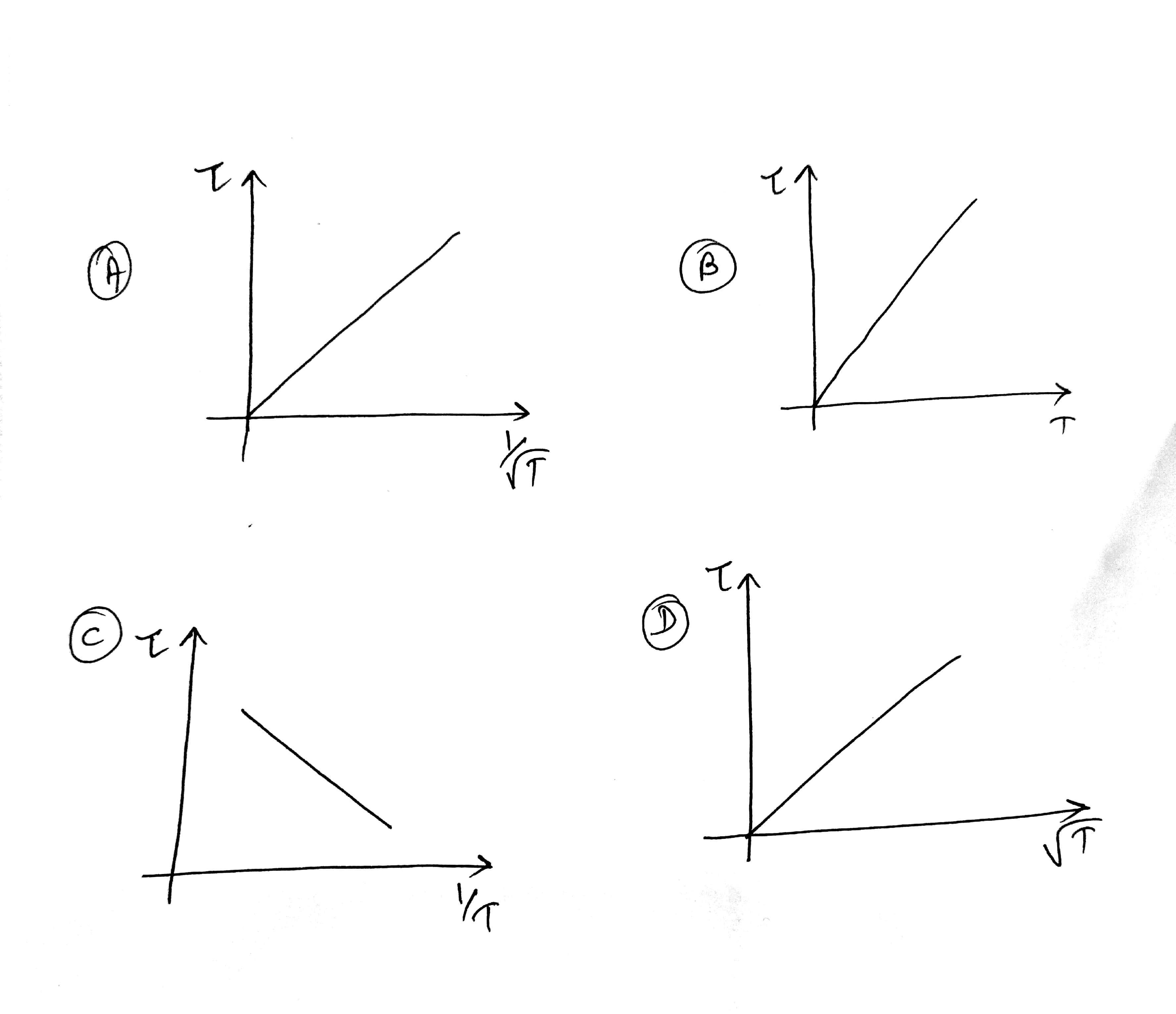Solution

As relaxation time is given as

where Y=mean free path

and

while

where N=number of molecules per unit volume

So keeping all the other quantities are constant.

we get

So the correct graph is given in option 1.

Q13. Critical angle for the medium with relative permittivity 3 and relative permeability .

Solution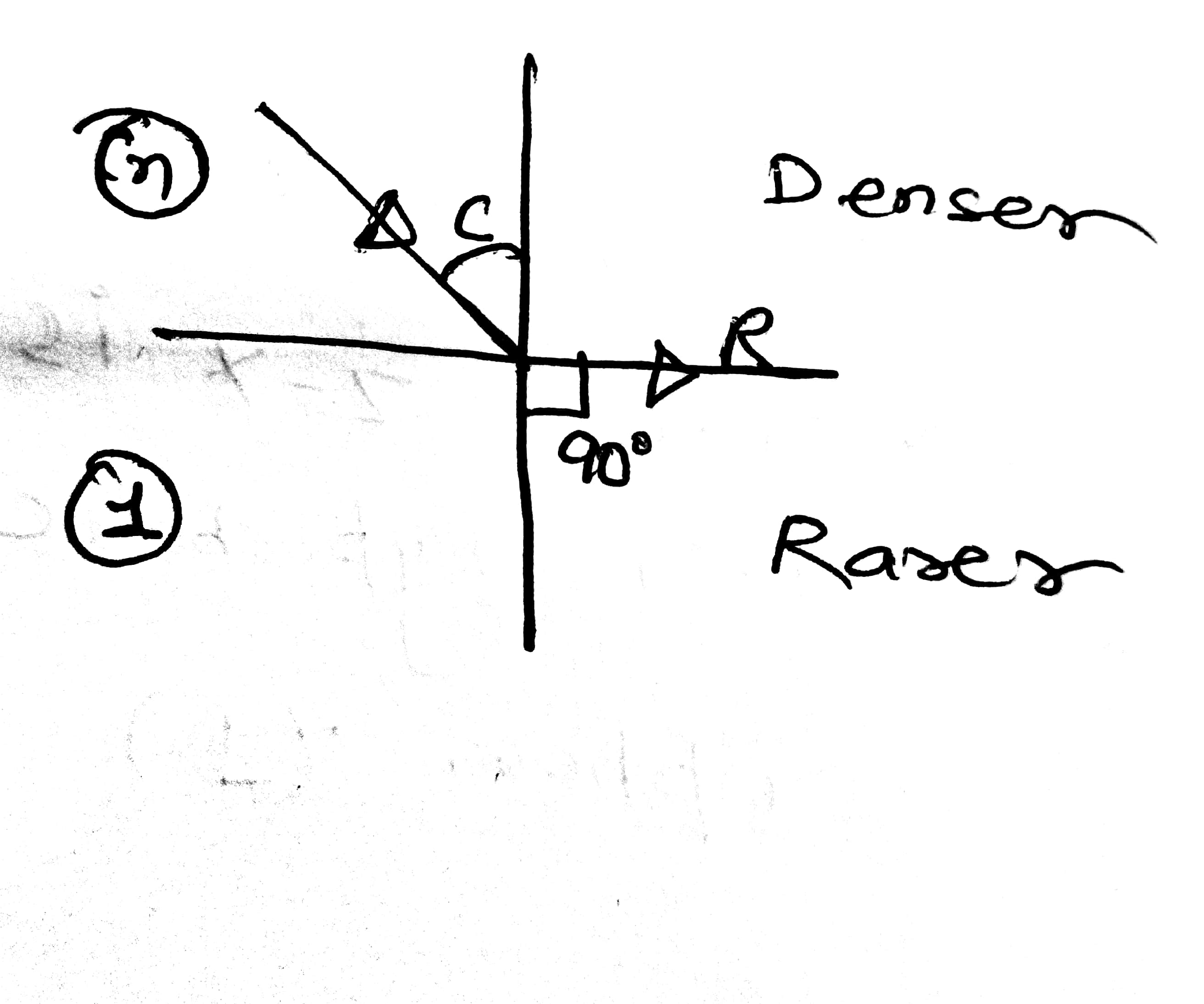and

By using snells law

Q14. A rod of mass 4m and length l was at rest on its perpendicular axis. Another particle of mass 'm', velocity u, collides at 45angle with its axis and stick at one ends. What is the angular velocity?

Solution: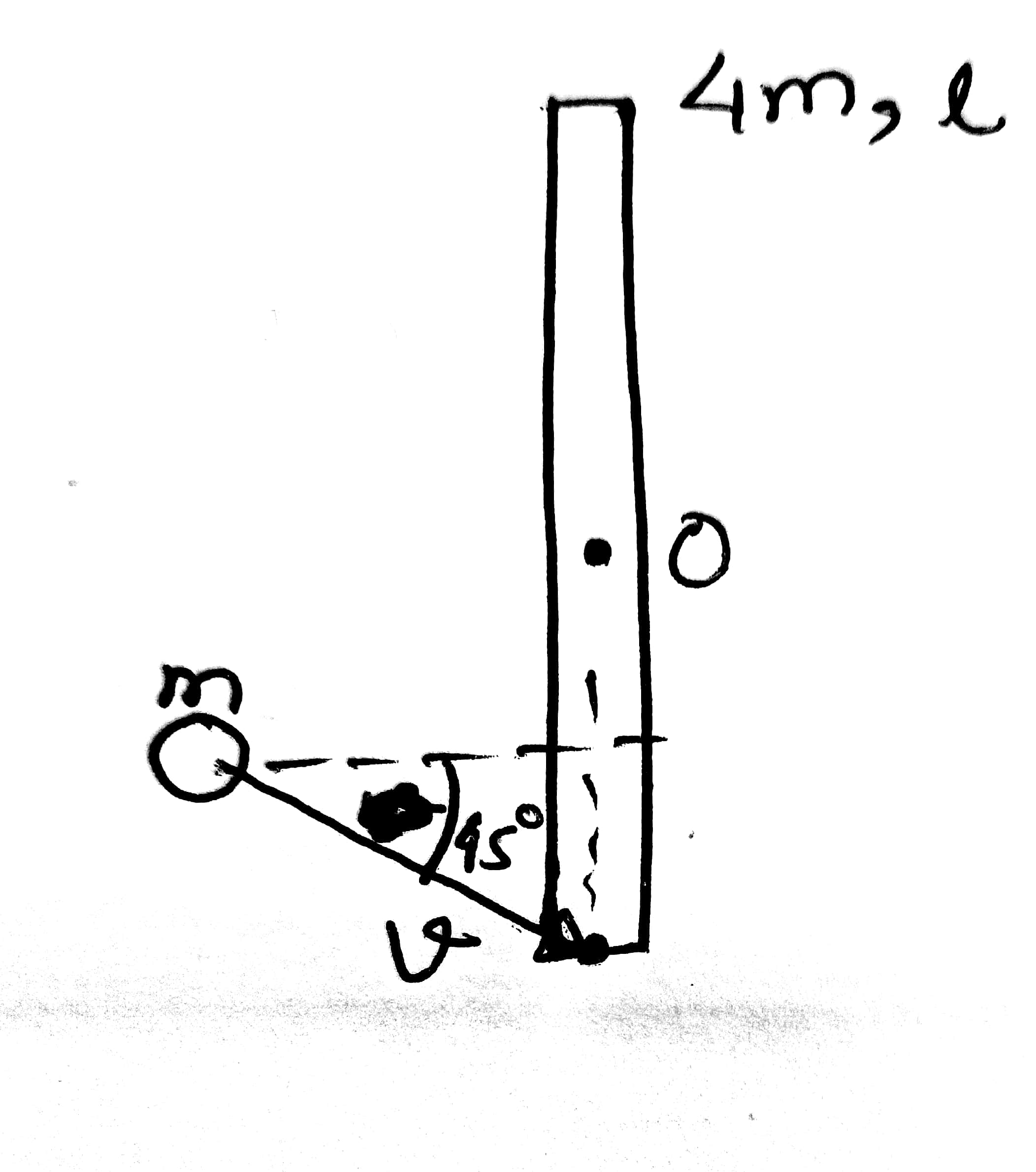Let us conserve angular momentum about O:-

So, , where  is linear momentum and  is the distance from centre O.

Now,

Here,

So,

Q15. What is the minimum density of the liquid, so that solid sphere of density can float?

Solution:-

Q-16 There are two metals A and B. Energy of incident photons are 4eV and 4.5 eV respectively. Maximum kinetic energy for ejected electron is for metal B. Relation between wavelength of ejected electron of A and B are Find work function of metal B.

Solution:-

As we know

So

Also

Using equation (1) and (2)

And using the Einstein equation

Q-17

A cylinder of height is floating in water at with height in air. Now temperature of water is raised to , height of cylinder in air becomes 21 cm. The ratio of density of water at to density of water at  is- (Consider expansion of cylinder is negligible)

Solution-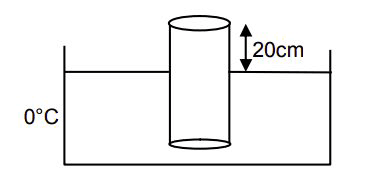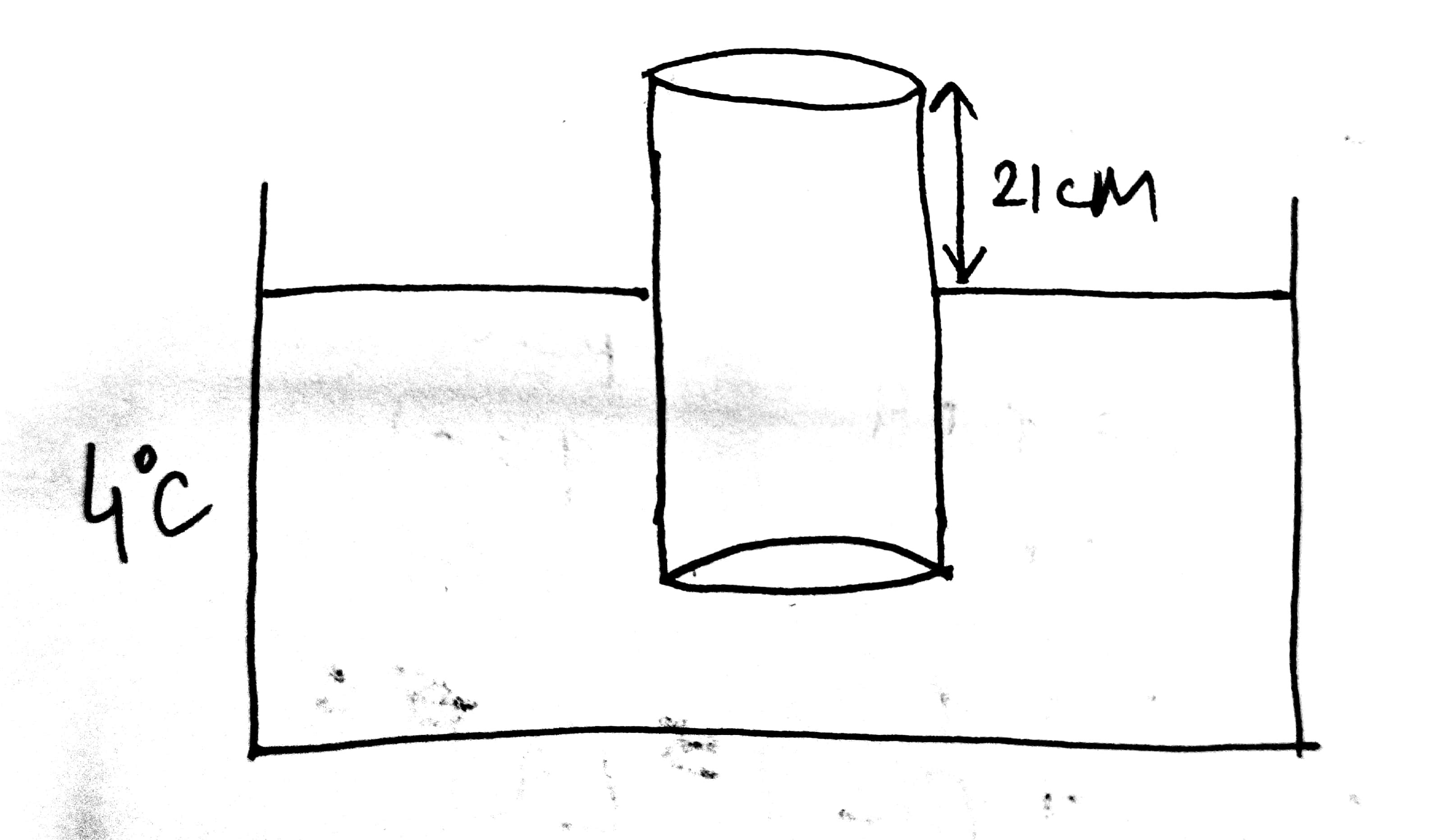Q-18 Number of the -particle deflected in Rutherford's -scattering experiment varies with the angle of deflection. Then the graph between the two is best represented by:-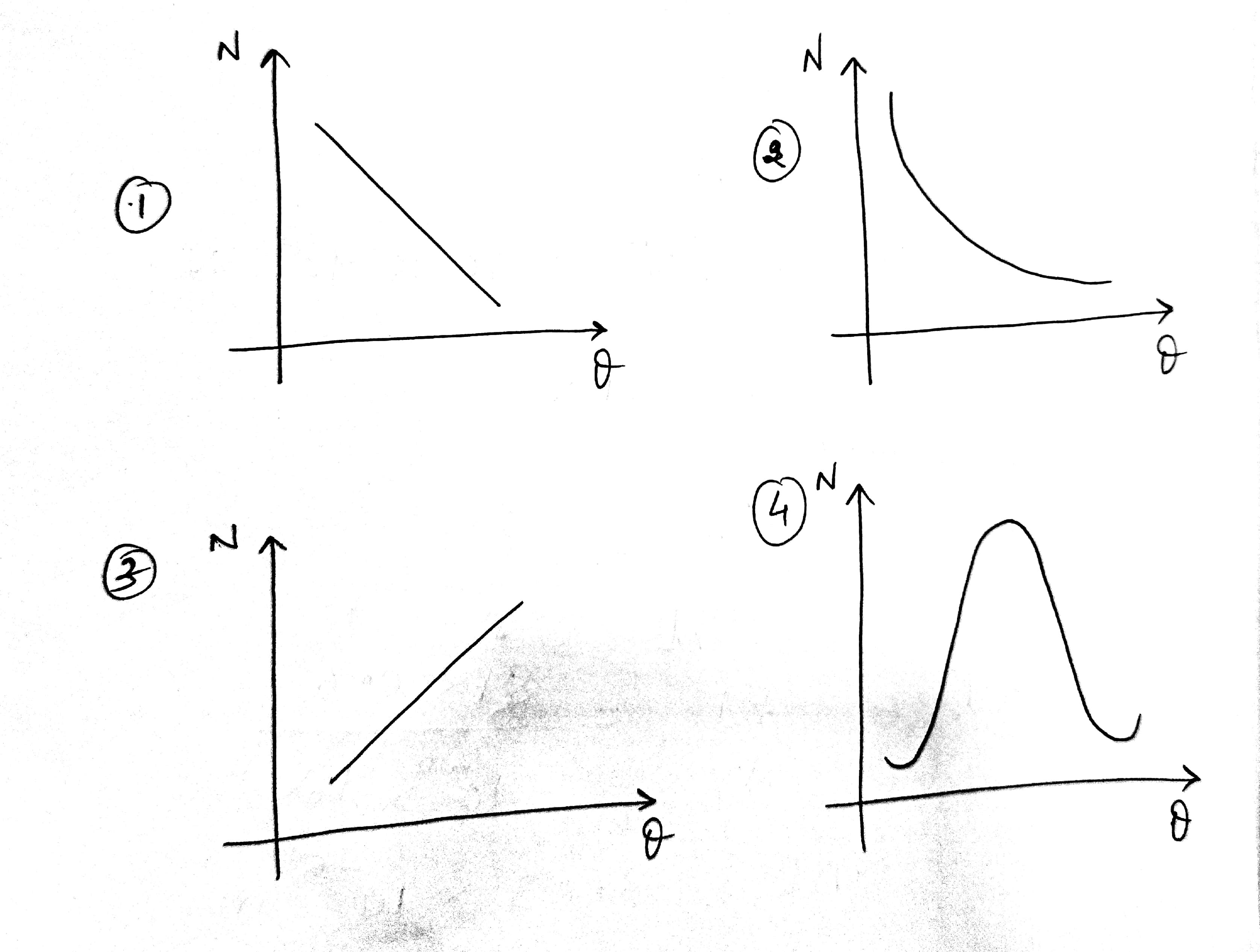Solution:-

Here when , Sin will be zero then N will tend to infinity.

and that is satisfied by only option 2

So option 2 is correct.

Q-19 The given loop is kept in a uniform magnetic field perpendicular to plane of loop. The field changes from 1000G to 500G in 5 seconds. The average induced emf in loop is-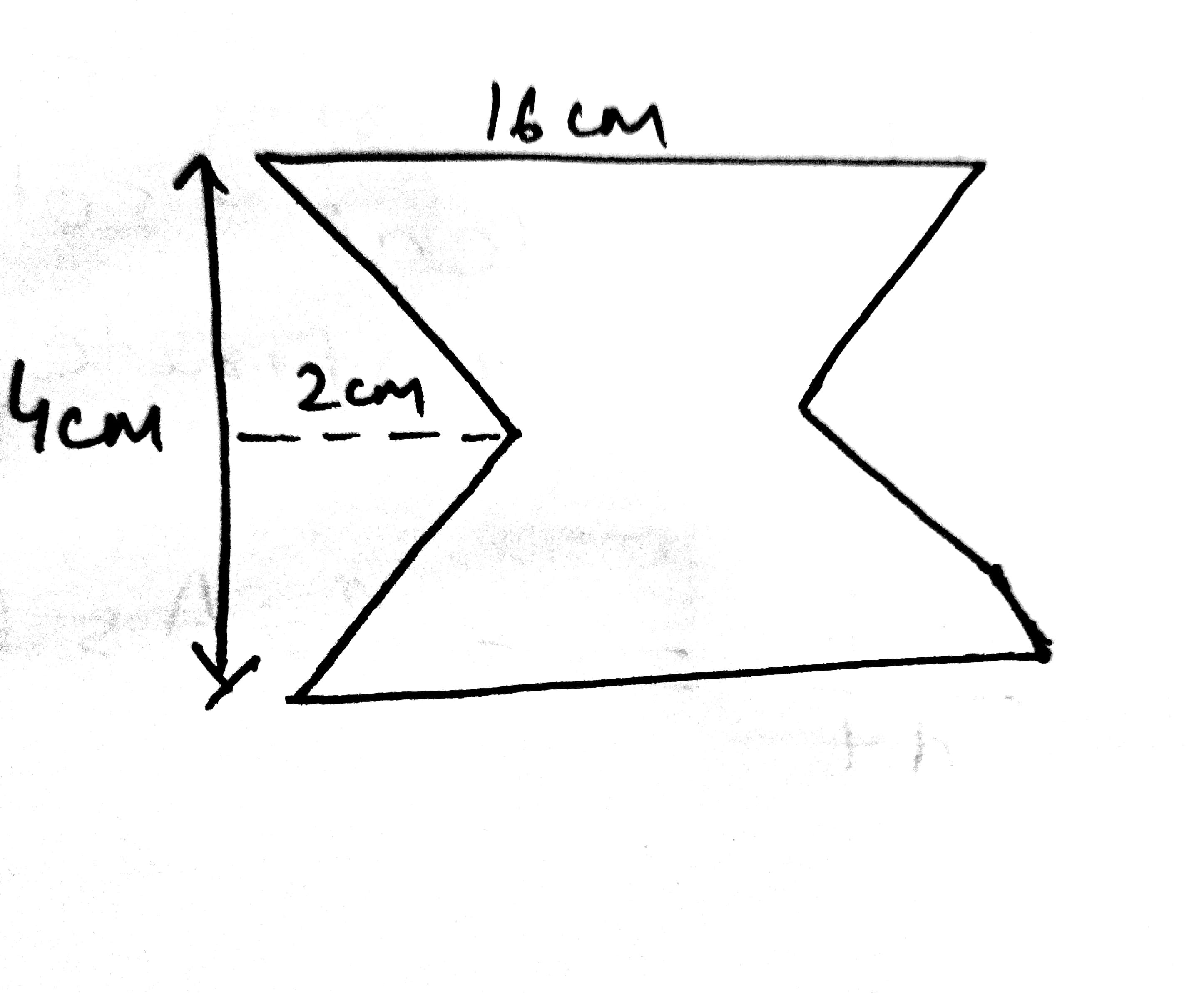(1)   (2)  (3)  (4)

Solution:-

Area of the given loop

Magnitude of emf

Q-20 Choose the correct Boolean expression for the given circuit diagram: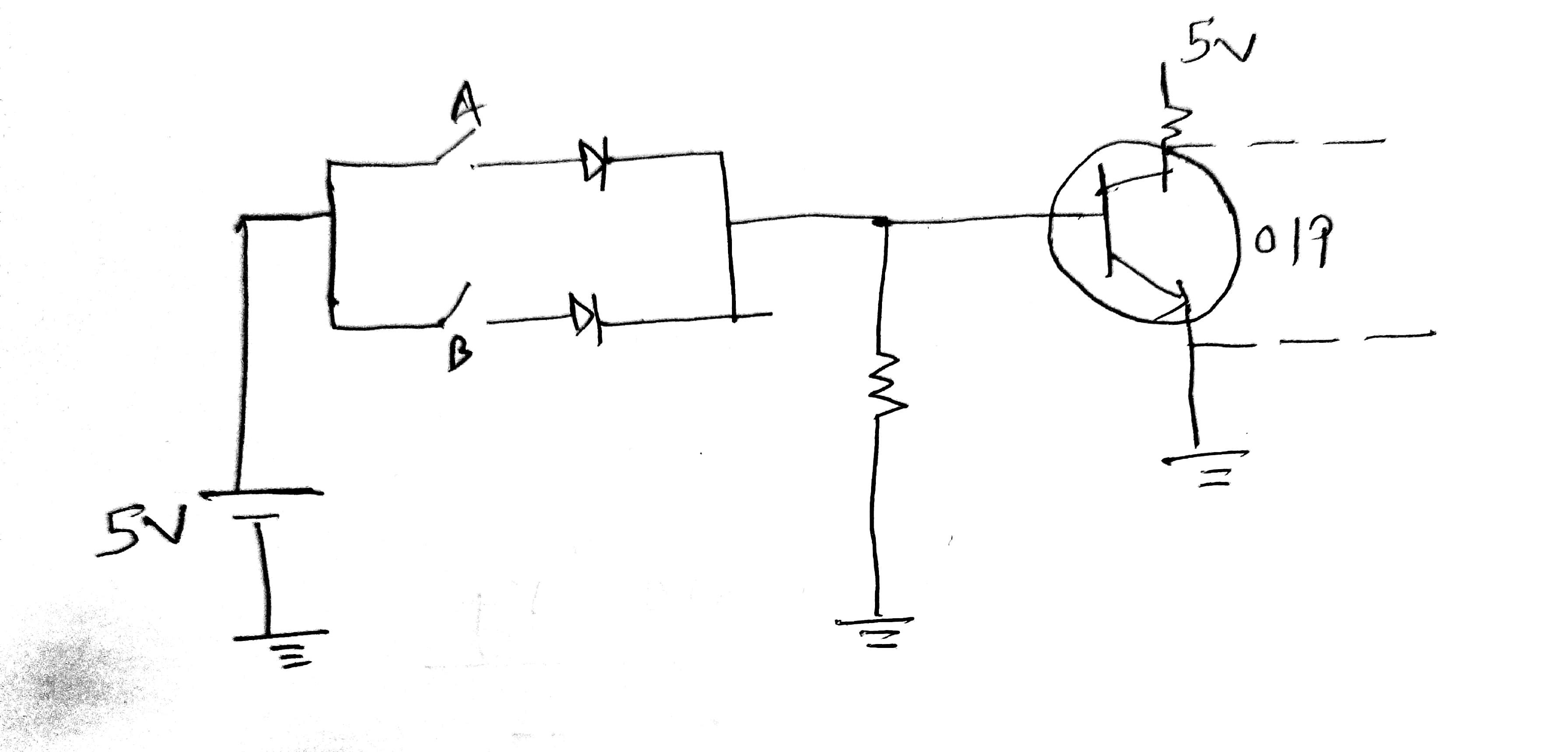Solution:-

The first part of the given circuit represents or gate whose out put is A+B

The second part of the circuit is NOT gate whose input is A+B and out put is

Q-21

Two masses each with mass 0.10kg are moving with velocities 3m/s along the x-axis and 5m/s along the y-axis respectively. After an elastic collision one of the mass moves with a velocity   . The energy of other mass after the collision is 10x then x is.

Sol-

For elastic collision

KEi = KEf

Q-22

Sol-

Q-23

An open organ pipe of length 1m contains a gas whose density is twice the density of the atmosphere at STP. Find the difference between fundamental and second harmonic frequencies if the speed of sound in the atmosphere is 300 m/s.

Sol-

Q-24 Two spherical bodies of mass are having radius respectively. The gravitational field of the two bodies with their radial distance is shown below. The value of is-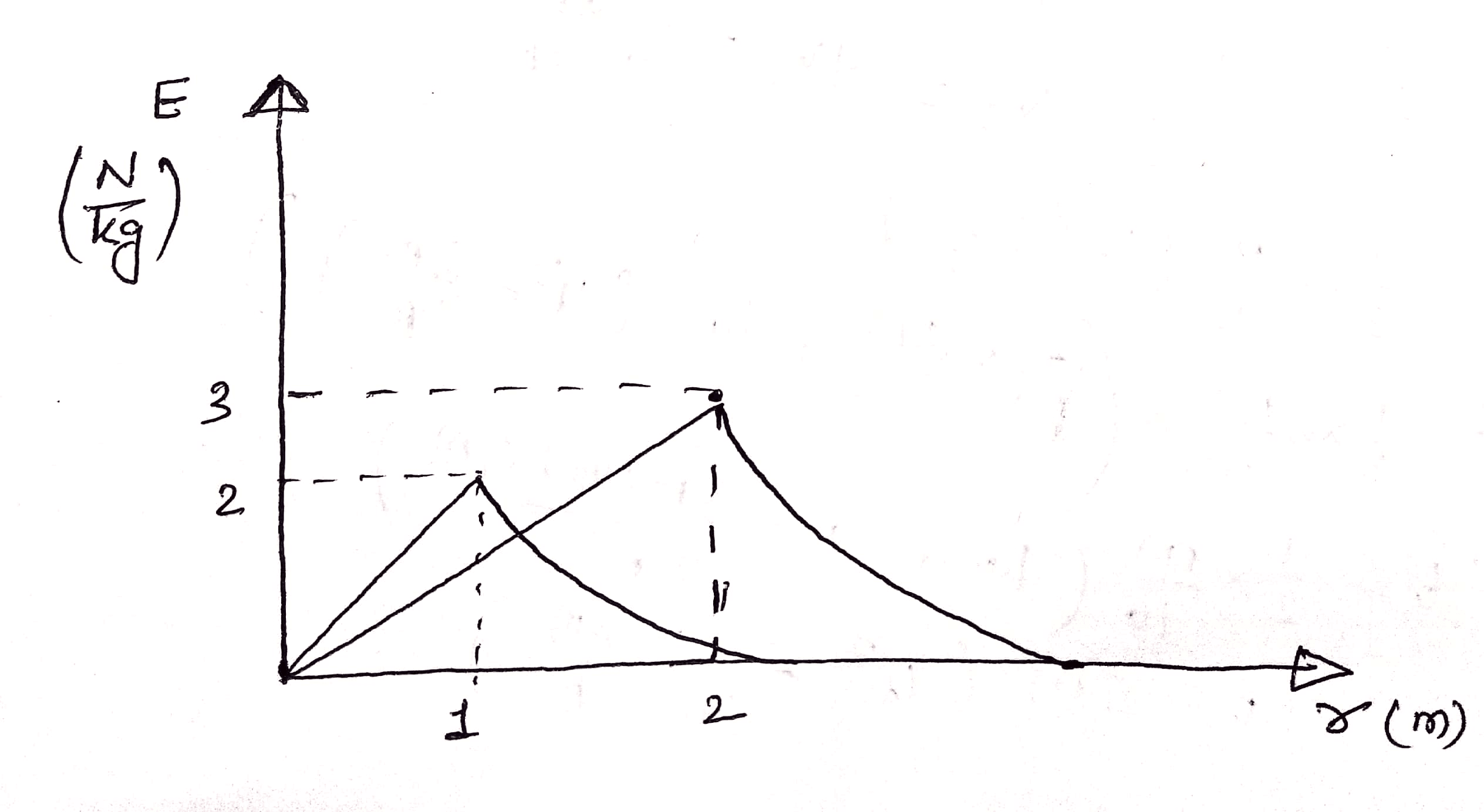Solution:-

Considering Case 2 and case 1, one by one

So, option 1 is correct.

Q-25

When proton of KE = 1.0 MeV moving in South to North direction enters the magnetic field (from West to East direction), it accelerates with a = 1012 m/s2 . The magnitude of magnetic field is–

Solution -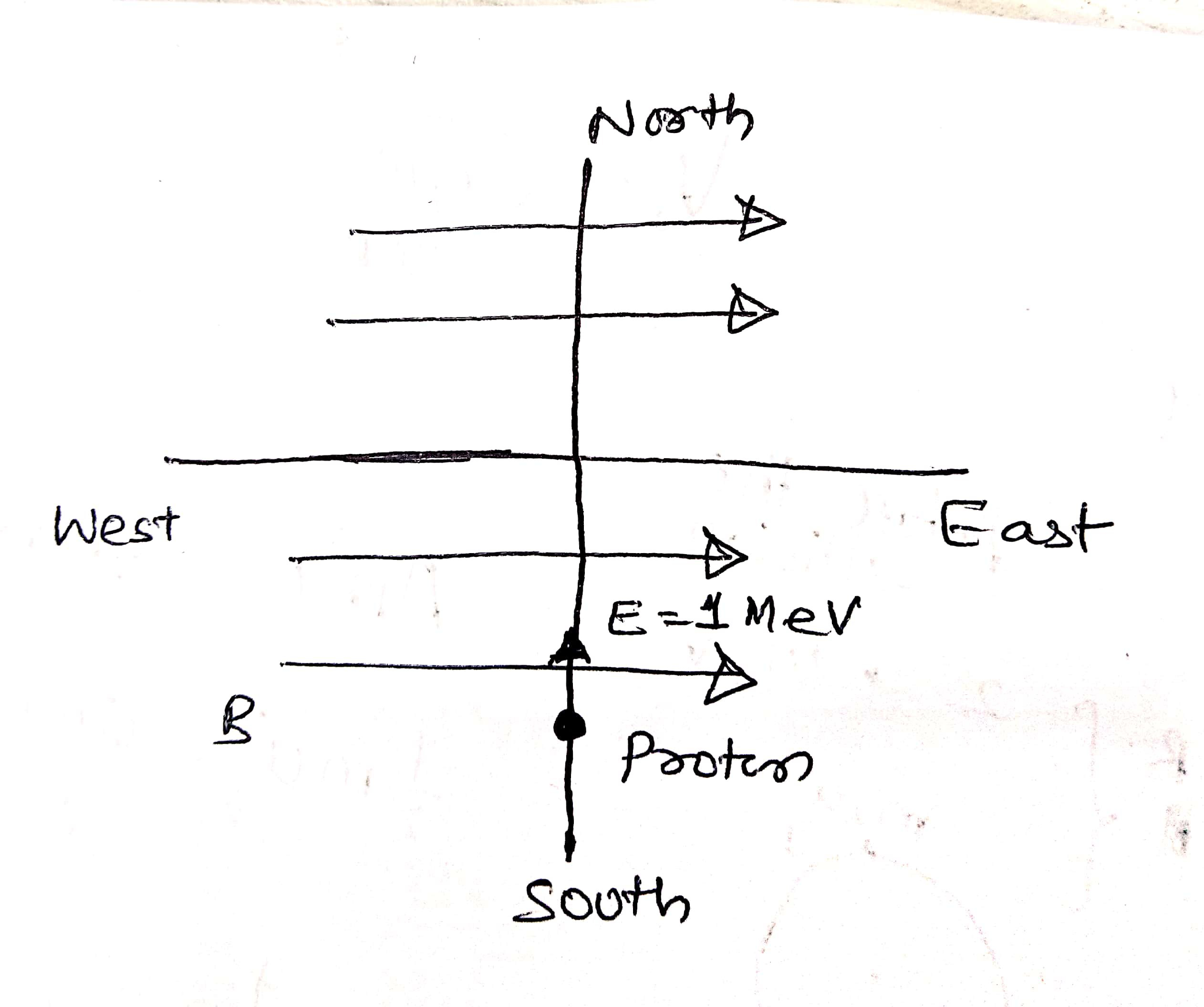So option (1) is correct